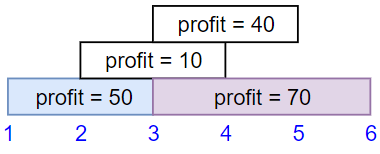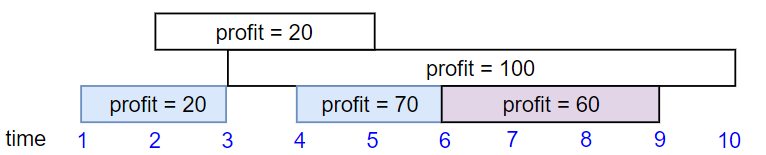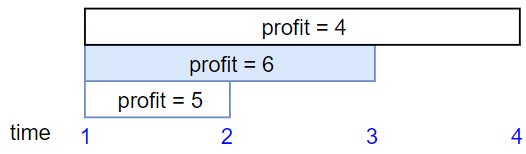## C# || Single-Threaded CPU – How To Find The Order CPU Will Process Tasks Using C#The following is a module with functions which demonstrates how to find the order CPU will process tasks using C#.

1. Get Order – Problem Statement

You are given n​​​​​​ tasks labeled from 0 to n – 1 represented by a 2D integer array tasks, where tasks[i] = [enqueueTimei, processingTimei] means that the i​​​​​​th​​​​ task will be available to process at enqueueTimei and will take processingTimei to finish processing.

You have a single-threaded CPU that can process at most one task at a time and will act in the following way:

• If the CPU is idle and there are no available tasks to process, the CPU remains idle.
• If the CPU is idle and there are available tasks, the CPU will choose the one with the shortest processing time. If multiple tasks have the same shortest processing time, it will choose the task with the smallest index.
• Once a task is started, the CPU will process the entire task without stopping.
• The CPU can finish a task then start a new one instantly.

Return the order in which the CPU will process the tasks.

Example 1:

``` Input: tasks = [[1,2],[2,4],[3,2],[4,1]] Output: [0,2,3,1] Explanation: The events go as follows: - At time = 1, task 0 is available to process. Available tasks = {0}. - Also at time = 1, the idle CPU starts processing task 0. Available tasks = {}. - At time = 2, task 1 is available to process. Available tasks = {1}. - At time = 3, task 2 is available to process. Available tasks = {1, 2}. - Also at time = 3, the CPU finishes task 0 and starts processing task 2 as it is the shortest. Available tasks = {1}. - At time = 4, task 3 is available to process. Available tasks = {1, 3}. - At time = 5, the CPU finishes task 2 and starts processing task 3 as it is the shortest. Available tasks = {1}. - At time = 6, the CPU finishes task 3 and starts processing task 1. Available tasks = {}. - At time = 10, the CPU finishes task 1 and becomes idle. ```

Example 2:

``` Input: tasks = [[7,10],[7,12],[7,5],[7,4],[7,2]] Output: [4,3,2,0,1] Explanation: The events go as follows: - At time = 7, all the tasks become available. Available tasks = {0,1,2,3,4}. - Also at time = 7, the idle CPU starts processing task 4. Available tasks = {0,1,2,3}. - At time = 9, the CPU finishes task 4 and starts processing task 3. Available tasks = {0,1,2}. - At time = 13, the CPU finishes task 3 and starts processing task 2. Available tasks = {0,1}. - At time = 18, the CPU finishes task 2 and starts processing task 0. Available tasks = {1}. - At time = 28, the CPU finishes task 0 and starts processing task 1. Available tasks = {}. - At time = 40, the CPU finishes task 1 and becomes idle. ```

2. Get Order – Solution

The following is a solution which demonstrates how to find the order CPU will process tasks.

``` 2. Get Order - Solution C# // ============================================================================ // Author: Kenneth Perkins // Date: Jan 27, 2023 // Taken From: http://programmingnotes.org/ // File: Solution.cs // Description: Demonstrates how to find the order CPU will process tasks // ============================================================================ public class Solution { private const int ENQUEUE_TIME = 0; private const int PROCESSING_TIME = 1; private const int INDEX = 2; public int[] GetOrder(int[][] tasks) { // Sort based on min task processing time or min task index. // Store enqueue time, processing time, task index. var nextTask = new PriorityQueue<int[], int[]>(Comparer<int[]>.Create((a, b) => (a[PROCESSING_TIME] != b[PROCESSING_TIME] ? (a[PROCESSING_TIME] - b[PROCESSING_TIME]) : (a[INDEX] - b[INDEX])))); // Store task enqueue time, processing time, index. int[][] sortedTasks = new int[tasks.Length][]; for (int index = 0; index < sortedTasks.Length; ++index) { sortedTasks[index] = new int; } for (int index = 0; index < tasks.Length; ++index) { sortedTasks[index][ENQUEUE_TIME] = tasks[index][ENQUEUE_TIME]; sortedTasks[index][PROCESSING_TIME] = tasks[index][PROCESSING_TIME]; sortedTasks[index][INDEX] = index; } Array.Sort(sortedTasks, (a, b) => a[ENQUEUE_TIME] - b[ENQUEUE_TIME]); int[] tasksProcessingOrder = new int[tasks.Length]; long currentTime = 0; int taskIndex = 0; int ansIndex = 0; // Stop when no tasks are left in array and heap. while (taskIndex < tasks.Length || nextTask.Count > 0) { if (nextTask.Count == 0 && currentTime < sortedTasks[taskIndex][ENQUEUE_TIME]) { // When the heap is empty, try updating currentTime to next task's enqueue time. currentTime = sortedTasks[taskIndex][ENQUEUE_TIME]; } // Push all the tasks whose enqueueTime <= currtTime into the heap. while (taskIndex < tasks.Length && currentTime >= sortedTasks[taskIndex][ENQUEUE_TIME]) { nextTask.Enqueue(sortedTasks[taskIndex], sortedTasks[taskIndex]); ++taskIndex; } int[] task = nextTask.Dequeue(); int processTime = task[PROCESSING_TIME]; int index = task[INDEX]; // Complete this task and increment currentTime. currentTime += processTime; tasksProcessingOrder[ansIndex++] = index; } return tasksProcessingOrder; } }// http://programmingnotes.org/ 123456789101112131415161718192021222324252627282930313233343536373839404142434445464748495051525354555657585960 // ============================================================================//    Author: Kenneth Perkins//    Date:   Jan 27, 2023//    Taken From: http://programmingnotes.org///    File:  Solution.cs//    Description: Demonstrates how to find the order CPU will process tasks// ============================================================================public class Solution {    private const int ENQUEUE_TIME = 0;    private const int PROCESSING_TIME = 1;    private const int INDEX = 2;     public int[] GetOrder(int[][] tasks) {        // Sort based on min task processing time or min task index.        // Store enqueue time, processing time, task index.        var nextTask = new PriorityQueue<int[], int[]>(Comparer<int[]>.Create((a, b) => (a[PROCESSING_TIME] != b[PROCESSING_TIME] ? (a[PROCESSING_TIME] - b[PROCESSING_TIME]) : (a[INDEX] - b[INDEX]))));         // Store task enqueue time, processing time, index.        int[][] sortedTasks = new int[tasks.Length][];        for (int index = 0; index < sortedTasks.Length; ++index) {            sortedTasks[index] = new int;        }        for (int index = 0; index < tasks.Length; ++index) {            sortedTasks[index][ENQUEUE_TIME] = tasks[index][ENQUEUE_TIME];            sortedTasks[index][PROCESSING_TIME] = tasks[index][PROCESSING_TIME];            sortedTasks[index][INDEX] = index;        }         Array.Sort(sortedTasks, (a, b) => a[ENQUEUE_TIME] - b[ENQUEUE_TIME]);        int[] tasksProcessingOrder = new int[tasks.Length];         long currentTime = 0;        int taskIndex = 0;        int ansIndex = 0;         // Stop when no tasks are left in array and heap.        while (taskIndex < tasks.Length || nextTask.Count > 0) {            if (nextTask.Count == 0 && currentTime < sortedTasks[taskIndex][ENQUEUE_TIME]) {                // When the heap is empty, try updating currentTime to next task's enqueue time.                currentTime = sortedTasks[taskIndex][ENQUEUE_TIME];            }             // Push all the tasks whose enqueueTime <= currtTime into the heap.            while (taskIndex < tasks.Length && currentTime >= sortedTasks[taskIndex][ENQUEUE_TIME]) {                nextTask.Enqueue(sortedTasks[taskIndex], sortedTasks[taskIndex]);                ++taskIndex;            }             int[] task = nextTask.Dequeue();            int processTime = task[PROCESSING_TIME];            int index = task[INDEX];             // Complete this task and increment currentTime.            currentTime += processTime;            tasksProcessingOrder[ansIndex++] = index;        }         return tasksProcessingOrder;    }}// http://programmingnotes.org/ ```

QUICK NOTES:
The highlighted lines are sections of interest to look out for.

The code is heavily commented, so no further insight is necessary. If you have any questions, feel free to leave a comment below.

Once compiled, you should get this as your output for the example cases:

``` [0,2,3,1] [4,3,2,0,1] ```

## C# || How To Determine If String Halves Are Alike Using C#The following is a module with functions which demonstrates how to determine if string halves are alike using C#.

1. Halves Are A like – Problem Statement

You are given a string s of even length. Split this string into two halves of equal lengths, and let a be the first half and b be the second half.

Two strings are alike if they have the same number of vowels (‘a’, ‘e’, ‘i’, ‘o’, ‘u’, ‘A’, ‘E’, ‘I’, ‘O’, ‘U’). Notice that s contains uppercase and lowercase letters.

Return true if a and b are alike. Otherwise, return false.

Example 1:

``` Input: s = "book" Output: true Explanation: a = "bo" and b = "ok". a has 1 vowel and b has 1 vowel. Therefore, they are alike. ```

Example 2:

``` Input: s = "textbook" Output: false Explanation: a = "text" and b = "book". a has 1 vowel whereas b has 2. Therefore, they are not alike. Notice that the vowel o is counted twice. ```

2. Halves Are A like – Solution

The following is a solution which demonstrates how to determine if string halves are alike.

``` 2. Halves Are A like - Solution C# // ============================================================================ // Author: Kenneth Perkins // Date: Jan 2, 2023 // Taken From: http://programmingnotes.org/ // File: Solution.cs // Description: Demonstrates how to determine if string halves are alike // ============================================================================ public class Solution { public bool HalvesAreAlike(string s) { // Get first half count int firstHalfVowel = 0; for (int index = 0; index < s.Length / 2; ++index) { if (IsVowel(s[index])) { ++firstHalfVowel; } } // Get second half count int secondHalfVowel = 0; for (int index = s.Length / 2 ; index < s.Length && secondHalfVowel <= firstHalfVowel; ++index) { if (IsVowel(s[index])) { ++secondHalfVowel; } } return firstHalfVowel == secondHalfVowel; } private static bool IsVowel(char ch) { ch = char.ToLower(ch); return ch == 'a' || ch == 'e' || ch == 'i' || ch == 'o' || ch == 'u'; } }// http://programmingnotes.org/ 1234567891011121314151617181920212223242526272829303132 // ============================================================================//    Author: Kenneth Perkins//    Date:   Jan 2, 2023//    Taken From: http://programmingnotes.org///    File:  Solution.cs//    Description: Demonstrates how to determine if string halves are alike// ============================================================================public class Solution {    public bool HalvesAreAlike(string s) {        // Get first half count        int firstHalfVowel = 0;        for (int index = 0; index < s.Length / 2; ++index) {            if (IsVowel(s[index])) {                ++firstHalfVowel;            }        }         // Get second half count        int secondHalfVowel = 0;        for (int index = s.Length / 2 ; index < s.Length && secondHalfVowel <= firstHalfVowel; ++index) {            if (IsVowel(s[index])) {                ++secondHalfVowel;            }        }        return firstHalfVowel == secondHalfVowel;    }     private static bool IsVowel(char ch) {        ch = char.ToLower(ch);        return ch == 'a' || ch == 'e' || ch == 'i' || ch == 'o' || ch == 'u';    }}// http://programmingnotes.org/ ```

QUICK NOTES:
The highlighted lines are sections of interest to look out for.

The code is heavily commented, so no further insight is necessary. If you have any questions, feel free to leave a comment below.

Once compiled, you should get this as your output for the example cases:

``` true false ```

## C# || How To Find The Maximum Profit In Job Scheduling Using C#The following is a module with functions which demonstrates how to find the maximum profit in job scheduling using C#.

1. Job Scheduling – Problem Statement

We have n jobs, where every job is scheduled to be done from startTime[i] to endTime[i], obtaining a profit of profit[i].

You’re given the startTime, endTime and profit arrays, return the maximum profit you can take such that there are no two jobs in the subset with overlapping time range.

If you choose a job that ends at time X you will be able to start another job that starts at time X.

Example 1:``` Input: startTime = [1,2,3,3], endTime = [3,4,5,6], profit = [50,10,40,70] Output: 120 Explanation: The subset chosen is the first and fourth job. Time range [1-3]+[3-6] , we get profit of 120 = 50 + 70. ```

Example 2:``` Input: startTime = [1,2,3,4,6], endTime = [3,5,10,6,9], profit = [20,20,100,70,60] Output: 150 Explanation: The subset chosen is the first, fourth and fifth job. Profit obtained 150 = 20 + 70 + 60. ```

Example 3:``` Input: startTime = [1,1,1], endTime = [2,3,4], profit = [5,6,4] Output: 6 ```

2. Job Scheduling – Solution

The following is a solution which demonstrates how to find the maximum profit in job scheduling.

``` 2. Job Scheduling - Solution C# // ============================================================================ // Author: Kenneth Perkins // Date: Jan 1, 2023 // Taken From: http://programmingnotes.org/ // File: Solution.cs // Description: Demonstrates how to find maximum profit in job scheduling // ============================================================================ public class Solution { public int JobScheduling(int[] startTime, int[] endTime, int[] profit) { if (startTime == null || startTime.Length == 0) { return 0; } var jobs = new List<Job>(); for (int index = 0; index < startTime.Length; ++index) { jobs.Add(new Job(startTime[index], endTime[index], profit[index])); } jobs.Sort((a, b) => a.Start - b.Start); return DFS(jobs, 0, new Dictionary<int, int>()); } private int DFS(List<Job> jobs, int currentJobIndex, Dictionary<int, int> maxProfits) { if (currentJobIndex >= jobs.Count) { return 0; } if (maxProfits.ContainsKey(currentJobIndex)) { return maxProfits[currentJobIndex]; } int next = BinarySearch(jobs, currentJobIndex); int inc = jobs[currentJobIndex].Profit + (next == -1 ? 0 : DFS(jobs, next, maxProfits)); int exc = DFS(jobs, currentJobIndex + 1, maxProfits); int maxProfit = Math.Max(inc, exc); maxProfits[currentJobIndex] = maxProfit; return maxProfit; } private int BinarySearch(List<Job> jobs, int currentJobIndex) { int lo = currentJobIndex; int high = jobs.Count - 1; int result = -1; while (lo <= high) { int mid = lo + (high - lo) / 2; if (jobs[currentJobIndex].End <= jobs[mid].Start) { result = mid; high = mid - 1; } else { lo = mid + 1; } } return result; } class Job { public int Start { get; set; } public int End { get; set; } public int Profit { get; set; } public Job(int startTime, int endTime, int profit) { Start = startTime; End = endTime; Profit = profit; } } }// http://programmingnotes.org/ 1234567891011121314151617181920212223242526272829303132333435363738394041424344454647484950515253545556575859606162636465666768697071 // ============================================================================//    Author: Kenneth Perkins//    Date:   Jan 1, 2023//    Taken From: http://programmingnotes.org///    File:  Solution.cs//    Description: Demonstrates how to find maximum profit in job scheduling// ============================================================================public class Solution {    public int JobScheduling(int[] startTime, int[] endTime, int[] profit) {        if (startTime == null || startTime.Length == 0) {            return 0;        }         var jobs = new List<Job>();        for (int index = 0; index < startTime.Length; ++index) {            jobs.Add(new Job(startTime[index], endTime[index], profit[index]));        }         jobs.Sort((a, b) => a.Start - b.Start);         return DFS(jobs, 0, new Dictionary<int, int>());    }     private int DFS(List<Job> jobs, int currentJobIndex, Dictionary<int, int> maxProfits) {        if (currentJobIndex >= jobs.Count) {            return 0;        }         if (maxProfits.ContainsKey(currentJobIndex)) {            return maxProfits[currentJobIndex];        }         int next = BinarySearch(jobs, currentJobIndex);        int inc = jobs[currentJobIndex].Profit + (next == -1 ? 0 : DFS(jobs, next, maxProfits));        int exc = DFS(jobs, currentJobIndex + 1, maxProfits);         int maxProfit = Math.Max(inc, exc);        maxProfits[currentJobIndex] =  maxProfit;        return maxProfit;    }     private int BinarySearch(List<Job> jobs, int currentJobIndex) {        int lo = currentJobIndex;        int high = jobs.Count - 1;        int result = -1;         while (lo <= high) {            int mid = lo + (high - lo) / 2;             if (jobs[currentJobIndex].End <= jobs[mid].Start) {                result = mid;                high = mid - 1;            } else {                lo = mid + 1;            }        }        return result;    }     class Job {        public int Start { get; set; }        public int End { get; set; }        public int Profit { get; set; }         public Job(int startTime, int endTime, int profit) {            Start = startTime;            End = endTime;            Profit = profit;        }    }}// http://programmingnotes.org/ ```

QUICK NOTES:
The highlighted lines are sections of interest to look out for.

The code is heavily commented, so no further insight is necessary. If you have any questions, feel free to leave a comment below.

Once compiled, you should get this as your output for the example cases:

``` 120 150 6 ```